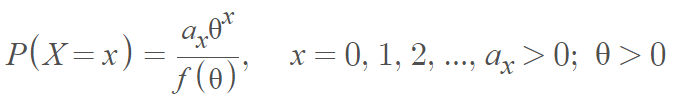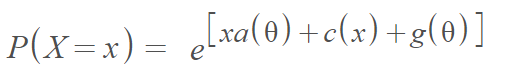# Power Series Distributions

Share on

Power series distributions are discrete probability distributions on a subset of natural numbers. The distributions are named because they are constructed from the power series. Many discrete distributions fall under this general category of distributions including the binomial distribution, negative binomial distribution, and Poisson distribution.

A discrete random variable X has a power series distribution given as:Where f(θ) is a generating function which is positive, finite, and differentiable .

The distribution is a member of the exponential family of distributions and can be expressed as follows :Where a and g are functions of θ, an unknown parameter and c is a function of x.

## Special Properties of Power Series Distributions

The power series distribution has some special properties:

 Condition Power Series Tends to… If θ = p / (1 – p); f(θ) = (1 + θ)n; s = {1, 2, 3, … n} Binomial distribution. If f(θ) = eθ and s = {0, 1, 2, 3, … ∞} Poisson Distribution. If θ = p / (1 – p); f(θ) = (1 + θ)-n; s = {0, 1, 2, 3, … ∞} Negative Binomial Distribution If f(θ) = -log (1 – θ) and s = {1, 2, …}, Logarithmic distribution

## Variations and Compounds of the Power Series Distribution

Several compounded distributions exist in the literature including the Weibull- power series distribution and the generalized Gompertz-power series distributions, obtained by compounding the generalized Gompertz distribution (a generalization of the exponential distribution) and the power series distributions .

Other variations include the exponential power series distribution, a composition of the exponential distribution with the power series distribution which gives a distribution with a decreasing failure rate .

## References

 Gupta, R. (1974). Modified Power Series Distribution and Some of Its Applications. The Indian Journal of Statistics, Volume 36, Series B, Pt. 3, pp. 288-298.
 Sakia, R. (2018). Application of the Power Series Probability Distributions for the Analysis of Zero-Inflated Insect Count Data. Open Access Library Journal, Vol.5 No.10.
 Tahmasebi, S. & Jafari, A. (2000). Generalized Gompertz-Power Series Distributions. Retrieved December 14, 2021 from: https://www.academia.edu/25858978/Generalized_Gompertz-Power_Series_Distributions
 Chahkandi, M. & Ganjali, M. (2009). On some lifetime distributions with decreasing failure rate. Computational Statistics & Data Analysis 53(12): 4433-4440.

CITE THIS AS:
Stephanie Glen. "Power Series Distributions" From StatisticsHowTo.com: Elementary Statistics for the rest of us! https://www.statisticshowto.com/power-series-distributions/
---------------------------------------------------------------------------Need help with a homework or test question? With Chegg Study, you can get step-by-step solutions to your questions from an expert in the field. Your first 30 minutes with a Chegg tutor is free!